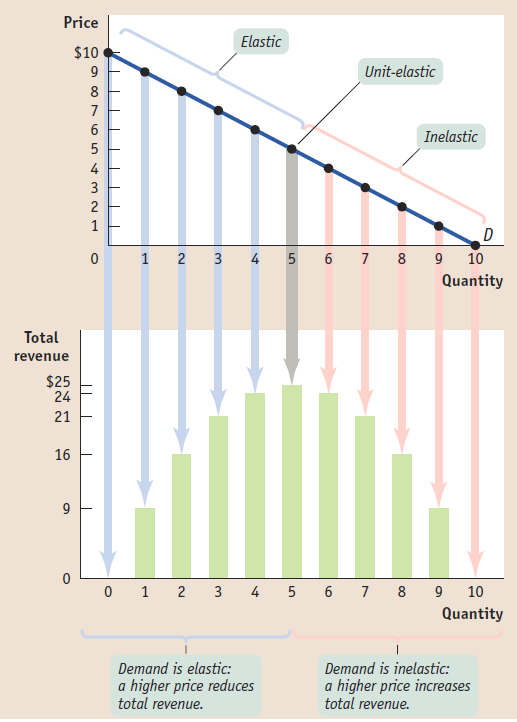Economics Tutors | Micro, Macro & Econometrics Tuitions | EconTutors

# London Economics Tutoring – Learn from LSE Kings UCL Economics Tutors

London Economics Tutor at EconTutors provides top tutors for in-person tutoring near London. Our tutors tailor lessons for A levels economics, IB economics, GCSE and AP economics. We hire from LSE, Kings, UCL, and Warwick. All our tutors have in-class teaching experience.### Total Revenue and Elasticity

A relationship between price elasticity and total revenue is shown in the figure above. Recall that total revenue is price x quantity. As price goes up, quantity demanded goes down. Thus, the effect on total revenue is unclear because if increase in price is more than off-set by decrease in quantity demanded, then total revenue will decrease.

If demand is relatively elastic then a decrease in price leads to a more than proportional increase in quantity demanded. This leads to an increase in total revenue. As can be seen in the graph below. On the other hand, when demand is relatively inelastic, a decrease in price leads to a less than proportional increase in quantity demanded. As a result, total revenue decreases.

In brief, total revenue is maximized when the elasticity of demand is unitary. In the graph above, that is at a price of 5. Consequently, if price falls or price rises from this price level, the total revenue will fall. To summarize, demand elasticity has a relationship with total revenue that depends on the size of the elasticity.

### Book a free lesson today with our London Economics tutor for your customized tutoring plan. Our tutors tailor tutoring plans for the following courses

• EC201 Microeconomics Principles tutor
• EC210 Macroeconomic Principles
• EC220 Introduction to Econometrics
• EC221 Principles of Econometrics
• EC230 Public Policy LSE
• MG205 Econometrics Theory and Applications LSE
• FM212 Principles of Finance LSE
• FM473 Financial Markets LSE
• FM474 Managerial Finance LSE
• 5SSMN224 Corporate Finance at King’s College
• 5SSPP213 Econometrics King’s College
• 6QQMN969 International Finance at King’s College
• 5SSPP221 Microeconomics at King’s College
• 4SSMN136 Principles of Economics at King’s College
• ECON2007 Quantitative Economics and Econometrics at UCL

Other popular courses:

Get Started

## See the #1 economics mentoring platform in action

Request a demo